Next: Advice Taking Scenario Up: Blocks World Example Previous: Theorem 1

#### Proof

The characterization of the semantics of NAT's in terms of second order logic theories including circumscription formulas and proposition 1 in [Lifschitz1995] allow us to prove the equivalence between the following axiom sets.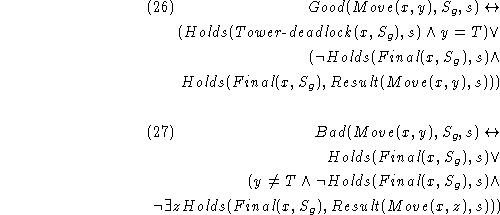Now, we use several rules for computing circumscription described in [Lifschitz1993]. The first equivalence below can be proved using formula (19) and proposition 2 in [Lifschitz1993]. The second equivalence uses formula (19) and proposition 3 in that paper.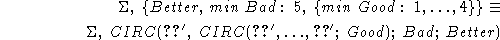Using the equivalence (27) in section 3.2 of [Lifschitz1993], we can prove that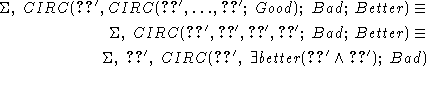is equivalent to the following formula, which does not depend on better.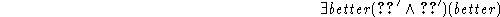Finally, predicate completion [Lifschitz1993] give us the result of the theorem.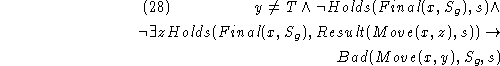Theorem 1 shows that the nested abnormality theory described byis equivalent to the second order theory whose axioms are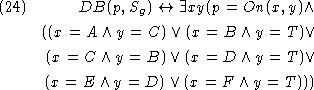,and. This means that using theorem proving methods for first order logicwe can decide the selectability of any situation with respect to the strategy described by axiomsto. For example, we can prove that action Move(A,T) is selectable at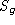(i.e.,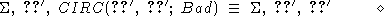), but action Move(C,T) is not (i.e.,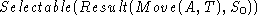).

Josefina Sierra
Tue Jul 21 09:54:27 PDT 1998